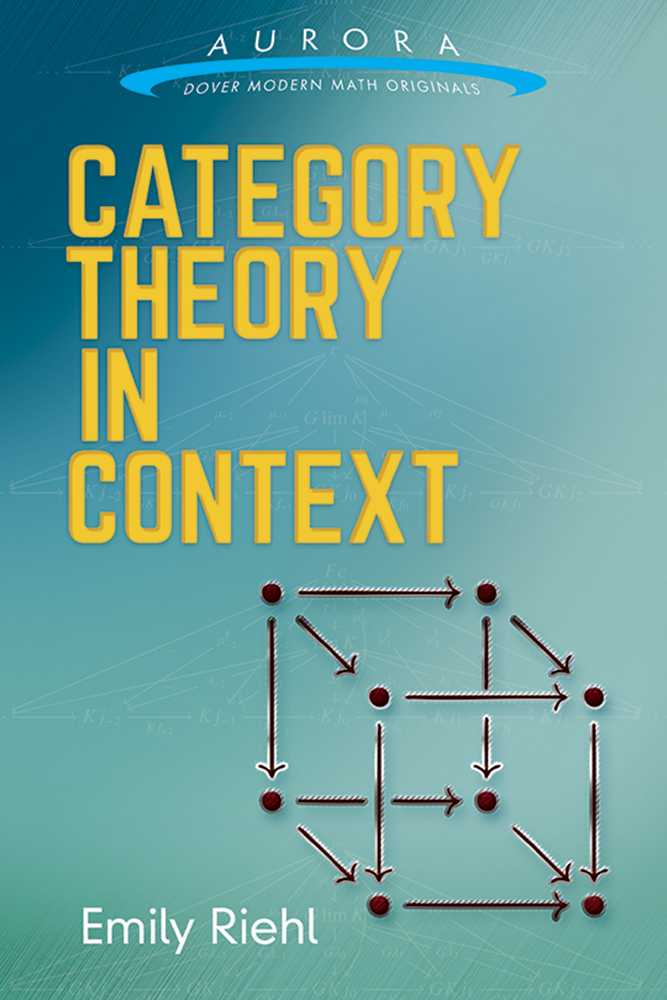## November 29, 2016

### Quarter-Turns

#### Posted by Tom LeinsterTeaching linear algebra this semester has made me face up to the fact that for a linear operator $T$ on a real inner product space, $\langle T x, x \rangle = 0 \,\, \forall x \,\, \iff \,\, T^\ast = -T$ whereas for an operator on a complex inner product space, $\langle T x, x \rangle = 0 \,\, \forall x \,\, \iff \,\, T = 0.$ In other words, call an operator $T$ a quarter-turn if $\langle T x, x \rangle = 0$ for all $x$. Then the real quarter-turns correspond to the skew symmetric matrices — but apart from the zero operator, there are no complex quarter turns at all.

Where in my mental landscape should I place these facts?

Posted at 11:14 PM UTC | Permalink | Followups (24)

### Linear Algebraic Groups (Part 8)

#### Posted by John BaezThe course proceeds apace, but my notifications here have slowed down as I become over-saturated with work.

In Part 8, I began explaining a bit of algebraic geometry. Following the general pattern of this course I took a quasi-historical approach, explaining some older ideas before moving on to newer ones. I’m afraid I never got to explaining schemes. That’s a tragedy, but hey—life is full of tragedies, nobody will notice this one. Affine schemes is all I had time for, despite the fact that I was discussing a lot of projective geometry. And before explaining affine schemes, it seemed wise to mention some earlier ideas and their defects.

Posted at 1:36 AM UTC | Permalink | Followups (2)

## November 16, 2016

### Category Theory in Context

#### Posted by Emily RiehlIn my final year at Harvard and again in my first year at Johns Hopkins, I had an opportunity to teach an advanced undergraduate/beginning graduate-level topics course entitled “Category Theory in Context.” Its aim was to provide a first introduction to the basic concepts of category theory — categories, functors, natural transformations, the Yoneda lemma, limits and colimits, adjunctions, monads, and Kan extensions — while simultaneously discussing the implications of these ideas in a wide variety of areas of mathematics on which category theory sheds light.

I thought teaching this course would provide a fun opportunity to collect as many examples of this kind as I could, for which I solicited widely — more about this below. This provided the impetus to write lecture notes. And now they have been published by Dover Publications in their new Aurora: Modern Math Originals series.I extremely grateful to Dover for granting me permission to host a free PDF copy of the book on my website. This version is in some sense even better than the published version, in that I have been able to correct a handful of typos that were discovered after the print version was already in press.

Posted at 10:28 PM UTC | Permalink | Followups (10)

## November 10, 2016

### Linear Algebraic Groups (Part 7)

#### Posted by John BaezOne of the less obvious but truly fundamental realizations in group theory is the importance of the ‘parabolic subgroups’ of a linear algebraic group. Today we’ll sneak up on this realization using the example of $\mathrm{GL}(n)$.

We’ve already seen the Klein geometry corresponding to this group has important kinds of figures — points, lines, planes, etc. — whose stabilizers are certain nice groups called ‘maximal parabolic subgroups’ of $\mathrm{GL}(n)$. But there are also important figures build from these, like ‘a point lying on a line’, or ‘a line lying on a plane’. These are called ‘flags’, and their stabilizers are called ‘parabolic subgroups’. Today we’ll work out what these parabolic subgroups of $\mathrm{GL}(n)$ are like. Especially important is the smallest one, called the ‘Borel’.

With this intuition in hand, we’ll want to generalize all these concepts to an arbitrary linear algebraic group. Amazingly, you can just hand someone such a group, and they can figure out the important kinds of geometrical figures in its Klein geometry, by determining its parabolic subgroups!

Posted at 2:13 AM UTC | Permalink | Followups (3)

## November 7, 2016

### Linear Algebraic Groups (Part 6)

#### Posted by John BaezWhen you’re doing math, if you ever want to keep things from getting too wispy and ethereal, it’s always good to count something. In fact, even if counting were good for nothing else — a strange counterfactual, that — mathematicians might have invented it for this purpose. It’s a great way to meditate on whatever structures one happens to be studying. It’s not the specific numbers that matter so much, it’s the patterns you find.

Last time we introduced Grassmannians as a key example of Klein’s approach to geometry, where each type of geometrical figure corresponds to a homogeneous space. Now let’s count the number of points in a Grassmannian over a finite field. This leads to a $q$-deformed version of Pascal’s triangle. Then, if we categorify the recurrence relation defining the $q$-binomial coefficients, we’ll understand the Bruhat cells for Grassmannians over arbitrary fields!

Posted at 1:00 AM UTC | Permalink | Followups (3)

## November 3, 2016

### Linear Algebraic Groups (Part 5)

#### Posted by John BaezNow let’s look at projective geometry from a Kleinian viewpoint. We’ll take the most obvious types of figures — points, lines, planes, and so on — and see which subgroups of $\mathrm{GL}(n)$ they correspond to. This leads us to the concept of ‘maximal parabolic subgroup’, which we’ll later generalize to other linear algebraic groups.

We’ll also get ready to count points in Grassmannians over finite fields. For that, we need the $q$-deformed version of binomial coefficients.